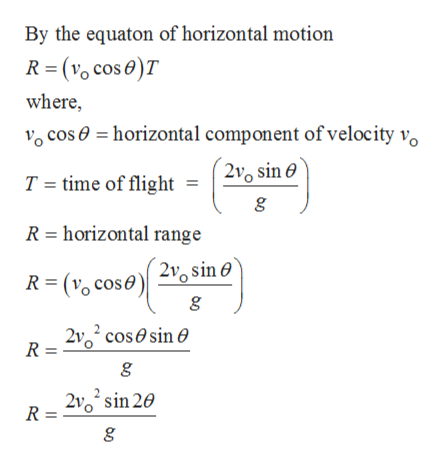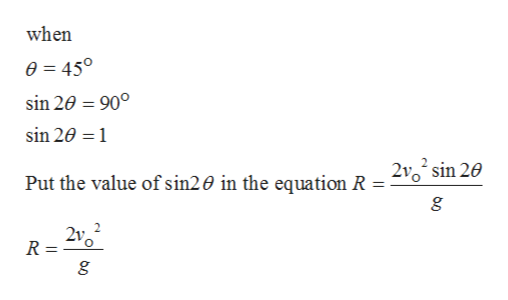what is the range of projectile? and what affect it?

Question

what is the range of projectile? and what affect it?

Step 1

When an object is thrown with an angle let say θ to the horizontal surface, it follows a curved path known as trajectory and the motion is called projectile motion. The object following projectile motion itself known as projectile.

Step 2

Horizontal distance covered by the object in time T is known as the range of the projectile motion. Here, blue line in the diagram shows the range of the projectile motion.help_outlineImage TranscriptioncloseBy the equaton of horizontal motion (vo cos e)T R where, cos horizontal component of velocity vo T = time of flight = 20 sine R horizontal range R( cose 2 sin 0' R = 2 cossin e R = 210 sin 20 fullscreen
Step 3

Horizontal range R is maximum whe...help_outlineImage Transcriptionclosewhen 0 450 sin 20 90° sin 20 1 Put the value of sin20 in the equation R = 210 sin 20 R = fullscreen

Want to see the full answer?

See Solution

Want to see this answer and more?

Our solutions are written by experts, many with advanced degrees, and available 24/7

See Solution
Tagged in

Physics